# Modelling of shear localization in solids by means of energy relaxation

## Abstract

An approach to the problem of shear localization is proposed. It is based on energy minimization principles associated with micro-structure developments. Shear bands are treated as laminates of first order. The micro-shear band is assumed to have a zero thickness, leading to an unbounded strain field and the special form of the energy within this micro-band. The energy is approximated by the mixture of potential of two low-strain and high-strain domains and it is non-convex. The problem of the non-convex energy arising due to the formation of shear bands is solved by energy relaxation in order to ensure that the corresponding problem is well-posed. An application of the proposed formulation to isotropic material is presented. The capability of the proposed concept is demonstrated through numerical simulation of a tension test.

## Background

Strain localization phenomena are observed in various materials as narrow zones of intense shearing, known as shear bands. In many cases, the formation of shear bands is accompanied by a softening response, characterized by a decrease in strength of the material with accumulated inelastic strain, often leading to complete failure ,. Therefore, research on formation of shear bands has received much attention.

In simulation of strain localization, mesh dependence is the direct consequence of the ill-posedness of the corresponding boundary value problem . Some enhanced continuum approaches can be found in literatures such as Cosserat theory-, nonlocal approaches,, and gradient-enhanced approach,. In these, an internal length scale is introduced to reflect certain small-scale effects assumed to be present in shear bands. The disadvantage of the corresponding numerical models is, however, that the element size is required be at least an order of magnitude smaller than the width of shear zones in order to obtain results independent of the mesh size .

The strong discontinuity approach, known as an alternative way to simulate strain localization without the introduction of characteristic lengths, rests upon the assumption that the displacement field is discontinuous -. This approach can be categorized into unregularized and regularized strong discontinuities. For unregularized strong discontinuities, the discontinuous displacement field induces an unbounded strain field having the character of a Dirac-delta distribution . For regularized strong discontinuity,, one considers a transition from continuous to discontinuous response by using an approximation of the Dirac-delta distribution. In both variants, however, it is necessary to determine the position of a shear band by tracking strong discontinuities.

Furthermore, another possibility to tackle the localization problem is the use of phenomenological plasticity frameworks, in which the shear band and its constitutive response are embedded in the macroscopic constitutive behavior. Pietruszczak and Xu , suggested a theoretical framework for the analysis of brittle materials. Constituent materials including the intact and localized zone are used to determine the average mechanical properties through homogenization technique. The constitutive equation in the region confined by the shear band involves the resultant force rate acting at the interface and the displacement discontinuity. Amero  suggested a procedure for incorporating localized small-scale effects of the material response in the large-scale problem, which is characterized by the standard local continuum. The large-scale regularization of rate-dependent models is accomplished with the formalism of strong discontinuities to model effectively the localized dissipation observed in localized failures of solids and structures. Nguyen et al. , presented an approach with enhanced kinematics to capture localized mode of deformation for quasi-brittle materials. The volume element intersected by a localization band is considered as a two-phase material. The continuity condition of the traction across the boundary of the localization boundary is enforced to couple two stresses corresponding to the behavior in the localization zone and the bulk elastic one.

In recent years a new methodology based on energy relaxation has been developed to simulate not only the development of material microstructures - but also localization phenomena in plasticity and damage -. For problems involving microstructure evolution and localization which is related to various local instability effects such as buckling, crashing, and cracking, integration of the stress-strain relation leads to a nonconvexity of the potential energy. This behaviour can be seen in many kinds of materials such as geomaterials, concrete, steel, composite. For detailed expositions of the different monotone stress-strain curves and the corresponding nonconvex energies consult . Dacorogna  showed that minimizers cannot be obtained in nonconvex variational problems. Instead, the quasiconvex envelope of the nonconvex energy, called the relaxed energy, should be studied to ensure the existence of minimizers. For the problem of strain localization, shear bands are treated as laminates of first order in microscopic level. The advantage of this theory, when applied to the problem at hand, is the natural formation of shear bands based on the energy minimization principles associated with micro-structure developments. In the works of Miehe and his coworkers -, the laminate orientation corresponding to a mode-II simple shear is approximated to the critical direction of non-convex energy based on the minimization of the determinant of the acoustic tensor. The width of a micro-shear band is finite. An incrementally variational formulation is based on an energy storage function and a dissipation function. Relaxation methods have been applied to crystal plasticity, see ,, and the references therein. However, the model in this paper is different in the sense as the direction of the shear band is variable, while in crystal plasticity, it is fixed.

Our model is based on the energy relaxation approach and aims to be applicable to any material which softens towards a critical state, for example geomaterials such as dense sands and over-consolidated clay. We treat shear bands as special laminates mixing two co-existing phases. When a shear band develops, the material at a point located inside the shear band is viewed as being decomposed into a high-strain and a low-strain domain. We will introduce specific potentials for the low strain and the high strain material behavior as depicted in Figure 1b.

This approach has some similarities with that one of Miehe and coworkers -. There, a non-convex potential obtained as condensed energy of an incremental variational approach is used. This leads to microstructures given as laminates of finite width. In our approach, we start from an energy given as the minimum of a low strain and a high strain potential where the latter one has linear growth only, while Miehe’s energy has superlinear growth. This leads to degenerated laminates which can be interpreted as true shear bands.

This work is based on the formulation introduced in . An application of the relaxation theory to linear elastic isotropic material and numerical simulations of a tension test under displacement control are shown. For inelastic materials, we assume that the elastic deformation is small compared to the inelastic deformation and can be neglected. A numerical example involving loading and unloading is studied in order to evaluate the performance of the proposed concept.

## Existence of solutions of non-linear boundary value problems and relaxation

The existence of equilibrium solutions of non-linear boundary value problems can be proved by employing the direct methods of calculus of variations. The basic idea of this method is the minimization of an energy functional. Let us consider the following total potential energy:

$\begin{array}{l}\Pi \left(\mathbit{u}\right)=\underset{\Omega }{\int }W\left(\mathbit{\epsilon }\right)\phantom{\rule{0.3em}{0ex}}\mathrm{d\Omega }-\underset{\Omega }{\int }\mathbit{u}·\mathbit{f}\phantom{\rule{0.3em}{0ex}}\mathrm{d\Omega }-\underset{\partial {\Omega }_{\sigma }}{\int }\mathbit{u}·\stackrel{̄}{\mathbit{t}}\phantom{\rule{0.3em}{0ex}}\mathit{\text{dA}}\phantom{\rule{1em}{0ex}}\end{array}$
(1)

where u is the displacement, f is the body force per unit volume, $\stackrel{̄}{\mathbit{t}}$ is the traction acting on the part Ω σ of the surface, and W is the nonlinear elastic strain energy. The strain field ε is given as the symmetric part of the displacement-gradient.

$\begin{array}{l}\mathbit{\epsilon }={\nabla }^{\mathrm{s}}\mathbit{u}.\end{array}$
(2)

Now we look at solutions of minimization problems of the form

(3)

For elastic materials, this corresponds to the well-known principle of minimum of the potential energy. But inelastic materials can be incorporated as well via a time-incremental formulation. In this case, W denotes the so-called condensed energy ,,.

However, for the case of negligible elastic deformations, inelastic materials can be treated in a completely analogous manner employing the theory established in ,. Fur this purpose, let us decompose the displacement gradient into rotation ω and total strain ε and the latter into its elastic and inelastic parts

$\begin{array}{l}\nabla \mathbit{u}=\omega +{\epsilon }_{\mathrm{E}}+{\epsilon }_{\mathrm{I}}.\end{array}$
(4)

If ε E is negligible, it follows

$\begin{array}{l}{\epsilon }_{\mathrm{I}}={\nabla }^{\mathrm{s}}\mathbit{u}.\end{array}$
(5)

Let a local dissipation functional for inelastic deformation be given by $\Delta \left({\stackrel{̇}{\mathbit{\epsilon }}}_{\mathrm{I}}\right)$, see ,. Then the total dissipation functional reads

$\begin{array}{l}D\left(\stackrel{̇}{\mathbit{u}}\right)=\underset{\Omega }{\int }\Delta \left({\stackrel{̇}{\mathbit{\epsilon }}}_{\mathrm{I}}\right)\phantom{\rule{0.3em}{0ex}}\mathrm{d\Omega }\end{array}$
(6)

while the total Gibbs energy is given by

$\begin{array}{l}G\left(\mathbit{u}\right)=-\underset{\Omega }{\int }\mathbit{u}·\mathbit{f}\phantom{\rule{0.3em}{0ex}}\mathrm{d\Omega }-\underset{\partial {\Omega }_{\sigma }}{\int }\mathbit{u}·\stackrel{̄}{\mathbit{t}}\phantom{\rule{0.3em}{0ex}}\mathrm{dA.}\end{array}$
(7)

The principle of minimum of the dissipation functional, , can now be formulated as

(8)

Because the terms $\left(-\underset{\Omega }{\int }\mathbit{u}·\stackrel{̇}{\mathbit{f}}\phantom{\rule{0.3em}{0ex}}\mathrm{d\Omega }-\underset{\partial {\Omega }_{\sigma }}{\int }\mathbit{u}·\stackrel{̇}{\stackrel{̄}{\mathbit{t}}}\phantom{\rule{0.3em}{0ex}}\mathit{\text{dA}}\right)$ occurring in $Ġ$ are not dependent on $\stackrel{̇}{\mathbit{u}}$ and thus do not enter the variation in (8), the functional in (8) can be equivalently replaced by

$\begin{array}{l}{\Pi }_{\mathrm{I}}\left(\stackrel{̇}{\mathbit{u}}\right)=\underset{\Omega }{\int }\Delta \left({\stackrel{̇}{\mathbit{\epsilon }}}_{\mathrm{I}}\right)\phantom{\rule{0.3em}{0ex}}\mathrm{d\Omega }-\underset{\Omega }{\int }\stackrel{̇}{\mathbit{u}}·\mathbit{f}\phantom{\rule{0.3em}{0ex}}\mathrm{d\Omega }-\underset{\partial {\Omega }_{\sigma }}{\int }\stackrel{̇}{\mathbit{u}}·\stackrel{̄}{\mathbit{t}}\phantom{\rule{0.3em}{0ex}}\mathrm{dA.}\end{array}$
(9)

The principle of minimum of the dissipation potential can now be reformulated as

(10)

Obviously the structure defined in (5), (9), (10) is completely analogous to that one given by (2), (1), (3) with u replaced by $\stackrel{̇}{u}$ and W(ε ) by $\Delta \left({\stackrel{̇}{\epsilon }}_{\mathrm{I}}\right)$.

From now on, we will focus our exposition on the elastic case keeping in mind that everything can be readily transferred to the inelastic case using the scheme explained above.

If the potential energy W is not quasiconvex in some region of the material body Ω, the functional

$\begin{array}{l}I\left(\mathbit{u}\right)=\underset{\Omega }{\int }W\left(\mathbit{\epsilon }\right)\phantom{\rule{0.3em}{0ex}}\mathrm{d\Omega }\phantom{\rule{1em}{0ex}}\end{array}$
(11)

is not sequentially weakly lower semicontinuous, thus the minimizer in problem $\left(\mathcal{P}\right)$ may be unattained . This is precisely the case for softening materials. As a result, numerical solutions suffer from discretization sensitivity . Following , the functional I(u ) may be replaced by a relaxed functional I Q (u )

$\begin{array}{l}{I}_{Q}\left(\mathbit{u}\right)=\underset{\Omega }{\int }\mathit{\text{QW}}\left(\mathbit{\epsilon }\right)\phantom{\rule{0.3em}{0ex}}\mathrm{d\Omega }\phantom{\rule{0.3em}{0ex}},\end{array}$
(12)

where the quasiconvexified functional Q W(ε ), also called quasiconvex envelope of W or quasiconvex hull of W, is defined by the minimization problem

$\begin{array}{l}\mathit{\text{QW}}\left(\mathbit{\epsilon }\right)=\underset{\mathbit{\phi }}{inf}\frac{1}{\omega }\underset{\omega }{\int }W\left(\mathbit{\epsilon }+{\nabla }^{\mathrm{s}}\mathbit{\phi }\right)\mathrm{d\Omega }\end{array}$
(13)

for a fixed but arbitrary bounded domain ω and every φ with φ =0 on ω, herein φ is denoted as fluctuation field. Using QW instead of W in $\left(\mathcal{P}\right)$ ensures the existence of minimizers . Let us now approximate QW by introducing specific fluctuation fields, so-called laminates, in Equation 13. We define a scalar function by

$\begin{array}{l}\psi \left(x\right)=\left\{\begin{array}{cc}\frac{x}{\xi },& 0\le x\le \xi ,\\ \frac{1-x}{1-\xi }& \xi \le x\le 1,\phantom{\rule{1em}{0ex}}\text{periodically repeated},\end{array}\right\\end{array}$
(14)

which is depicted in Figure 2a. The fluctuation field is defined by

$\begin{array}{l}\mathbit{\phi }\left(\mathbit{x}\right)=\mathbit{a}\psi \left(\mathbit{n}·\mathbit{x}\right),\phantom{\rule{1em}{0ex}}∥\mathbit{n}∥=1\end{array}$
(15)

corresponding to the laminate depicted in Figure 2b, where n is the unit normal vector to laminates and a is an arbitrary vector. Then the gradient of the fluctuation field φ has the following values:

$\begin{array}{l}\nabla \mathbit{\phi }\left(\mathbit{x}\right)=\left\{\begin{array}{cc}\frac{1}{\xi }\mathbit{a}\otimes \mathbit{n},& \text{if}\phantom{\rule{0.3em}{0ex}}\phantom{\rule{0.3em}{0ex}}\mathbit{x}\phantom{\rule{0.3em}{0ex}}\phantom{\rule{0.3em}{0ex}}\text{belongs to region 1},\\ -\frac{1}{1-\xi }\mathbit{a}\otimes \mathbit{n},& \text{if}\phantom{\rule{0.3em}{0ex}}\phantom{\rule{0.3em}{0ex}}\mathbit{x}\phantom{\rule{0.3em}{0ex}}\phantom{\rule{0.3em}{0ex}}\text{belongs to region 2}.\end{array}\right\\end{array}$
(16)

Without restriction we consider a representative volume element as shown in Figure 2b. Then the definition of quasiconvexified energy (13) reduces to

(17)

which can be written in the alternative form

(18)

where ξ1 and ξ2, respectively, are two volume fractions of the regions 1 and 2; ε1 and ε2, respectively, are the strain fields within regions 1 and 2.

In Equation 17, or equivalently 18, we find the definition of the so-called first order lamination hull ,. This is nothing more than quasiconvexification restricted to first-order laminates as possible fluctuation fields. The formulation proposed in this paper is developed based on that very notion.

## Shear bands as special laminates

In this paper, localization phenomena are regarded as microstructure developments associated with nonconvex potentials. We assume that the micro-structures consist of two domains: a low-strain domain and a high-strain one. Let us consider a representative volume element (RVE) obtained by zooming in on the region around point A as shown in Figure 3. The RVE is split into two volume fractions: the volume fraction ξ of the high-strain domain and the volume fraction (1−ξ) of the low-strain domain.

When strain localization occurs, the potential (energy) inside the shear band W2 is assumed to satisfy

$\begin{array}{l}{W}_{2}\left(\xi \mathbit{\epsilon }\right)=\left|\xi \right|{W}_{2}\left(\mathbit{\epsilon }\right).\end{array}$
(19)

For example, W2(ε ) may be taken in the following form

$\begin{array}{lcr}{W}_{2}\left(\mathbit{\epsilon }\right)& =& {\left(\mathbit{\epsilon }:\mathsc{D}:\mathbit{\epsilon }\right)}^{\frac{1}{\alpha }},\end{array}$
(20)

α=2, where $\mathsc{D}$ is symmetric fourth-order, positive definite tensor. For α=1, this energy corresponds to a linear-elastic material with elastic stiffness tensor given by $\mathsc{D}$. For varying α, it behaves more or less stiff in a nonlinear way.

According to the assumption above, the potential inside a shear band is positive homogeneous of first-order in the strain field (19). We will see later on, that only for this very form of the potential as given in Equation 20 corresponding to α=2, it has the desired property leading to strong discontinuities. If α is smaller than 2, the material will exhibit only weak discontinuities. If α is larger than 2, a relaxed energy does not exist because of lack of coercivity.

Based upon these considerations, let us start with the consideration of a very simple one-dimensional model to discuss the physical implications of the proposed approach. Then it will be generalized to two dimensions.

## One-dimensional problem

### Micro-strain

Shear bands are treated as special laminates mixing two co-existed phases (Figure 3). The volume fraction ξ characterizing the width of the micro-band may be defined by the ratio between the length scale and a characteristic geometric parameter ,. If the volume fraction ξ is finite (0<ξ<1), shear bands are represented as weak discontinuity. In this case, let us denote by ε1 the strain present outside and by ε2 the strain present within a micro-shear band (see Figure 1a). A visualization given in Figure 1a depicts the shape of a non-convex potential W and its convexification.

The volume fraction ξ of the micro-band varies between 0 and 1. Let us assume that the volume fraction ξ is rather small in comparison to the volume fraction of the RVE, then, the latter case does not happen. If ξ tends to zero, the micro-strain ε2 of the high-strain domain is unbounded. Then, a potential W responsible for a strong discontinuity (ξ→0) is depicted in Figure1b.

To clarify why ε2 is unbounded, let us start with the relation between the macro-strain ε and the two micro-strains ε1, ε2

$\begin{array}{l}\epsilon =\left(1-\xi \right){\epsilon }_{1}+\xi \phantom{\rule{0.3em}{0ex}}{\epsilon }_{2}.\end{array}$
(21)

Following Lambrecht and Miehe , we assume that

$\begin{array}{lcr}{\epsilon }_{1}& =& \epsilon -\xi \phantom{\rule{0.3em}{0ex}}d,\end{array}$
(22)
$\begin{array}{lcr}{\epsilon }_{2}& =& \epsilon +\left(1-\xi \right)\phantom{\rule{0.3em}{0ex}}d=\epsilon -\xi \phantom{\rule{0.3em}{0ex}}d+d,\end{array}$
(23)

where 0≤ξ≤1. Let us consider here the case d≥0 and ε≥0. In the case d≤0 and ε≤0, the procedure is completely similar. The case ε d<0 leading to |ε2|<|ε| does not exist. In order to analyze the limit ξ→0, we have to introduce an appropriately rescaled variable. Let us define s by

$\begin{array}{l}s=\mathrm{\xi d.}\end{array}$
(24)

Substituting (24) into Equations 23 and 23 yields

$\begin{array}{lcr}{\epsilon }_{1}& =& \epsilon -s,\end{array}$
(25)
$\begin{array}{lcr}{\epsilon }_{2}& =& \epsilon -s+\frac{s}{\xi }·\end{array}$
(26)

Let ξ tend to zero for fixed s. Then $\frac{s}{\xi }$ will grow out of bounds in comparison with (εs). Thus, Equation 26 can be simplified as

$\begin{array}{l}{\epsilon }_{2}\approx \frac{s}{\xi }\to \infty \phantom{\rule{1em}{0ex}}\text{as}\phantom{\rule{1em}{0ex}}\xi \to 0\phantom{\rule{0.3em}{0ex}}.\end{array}$
(27)

The assumption of a zero-width micro-shear band immediately leads to an unbounded strain (27) within the high-strain domain of the micro-shear band.

### Relaxed energy

Based on the values of the two micro-strains ε1 and ε2 the potential W can be divided into three domains (see Figure 1b). The quadratic part, denoted as W1, is the potential representing the behaviour at small strains. The linear part W2 is the potential representing the behaviour at very large strains. The energy W in the domain in strain space where W(ε )≠W1(ε ) and W(ε )≠W2(ε ) is of no importance since it does not influence the relaxed energy. Hence, using (25) and (27), we can formulate a mixture potential of the two energies as

$\begin{array}{l}{W}^{\text{mix}}\left(\epsilon \right)=\left(1-\xi \right){W}_{1}\left({\epsilon }_{1}\right)+\xi {W}_{2}\left({\epsilon }_{2}\right)=\left(1-\xi \right){W}_{1}\left(\epsilon -s\right)+\xi {W}_{2}\left(\frac{s}{\xi }\right).\end{array}$
(28)

Based on the assumption (20) with the special case $\mathsc{D}={A}^{2}\mathsc{I}$, it can be simplified as

$\begin{array}{lcr}{W}^{\text{mix}}\left(\epsilon \right)& =& {W}_{1}\left(\epsilon -s\right)+A\left|s\right|.\end{array}$
(29)

It should be noted that the classical Hencky model of deformation - bears a strong relation to our theory. To this end, the energy (29) can be viewed a composed of the elastic energy W1(εs) and the dissipated energy A|s|. The displacement u and the plastic strain s can then be determined by the following minimization problem

$\begin{array}{l}\underset{\text{inf}}{}\left\{\underset{\Omega }{\int }{W}^{\text{mix}}\left(\epsilon \left(u\right)-s\right)\phantom{\rule{0.3em}{0ex}}\mathrm{d\Omega }-\underset{\Omega }{\int }u·f\phantom{\rule{0.3em}{0ex}}\mathrm{d\Omega }-\underset{\partial {\Omega }_{\sigma }}{\int }u·\stackrel{̄}{t}\phantom{\rule{0.3em}{0ex}}\mathit{\text{dA}}\phantom{\rule{1em}{0ex}}\phantom{\rule{1em}{0ex}}\left|\rightu,s\right\},\end{array}$
(30)

where Wmix is refered to in the literature as the elasto-plastic superpotential . In the plastic regime, the stress state lies on the yield surface, indicating that σ=A is in agreement with Equation 34.

The non-convex mixture potential (29) gives rise to an ill-posed boundary value problem making the calculation of shear bands dependent on the particularities of the numerical discretization used. By introducing the concept of relaxation the problem can be resolved and becomes well-posed. The relaxed potential is obtained by the minimization procedure

$\begin{array}{l}{W}_{R}\left(\epsilon \right)=\underset{\text{inf}}{}\left\{{W}^{\text{mix}}\left(\epsilon \right)\phantom{\rule{1em}{0ex}}\left|\rights\right\}.\end{array}$
(31)

As mentioned in section ‘Micro-strain’ we consider here the case d≥0 and ε≥0. Then the relaxed potential (31) can be rewritten as follows:

$\begin{array}{l}{W}_{R}\left(\epsilon \right)=\underset{\text{inf}}{}\left\{{W}^{\text{mix}}\left(\epsilon \right)\phantom{\rule{1em}{0ex}}\left|\rights\phantom{\rule{0.3em}{0ex}},\phantom{\rule{0.3em}{0ex}}s\ge 0\right\}.\end{array}$
(32)

The corresponding stress is obtained by taking the derivative of the mixture potential (28) with respect to ε

$\begin{array}{l}\sigma \left(\epsilon \right)=\left(1-\xi \right)\sigma \left({\epsilon }_{1}\right)+\mathrm{\xi \sigma }\left({\epsilon }_{2}\right).\end{array}$
(33)

The unique stationary point of Equation 32 can be obtained from

$\begin{array}{l}\sigma \left(\epsilon \right)=\sigma \left({\epsilon }_{1}\right)=\sigma \left({\epsilon }_{2}\right)=\mathrm{A.}\end{array}$
(34)

The slope of the relaxed potential represented by (34), i.e. at values of ε where it differs from W1, is constant, consequently, the relaxed tangent modulus is equal to zero. Here, the material parameter A can be interpreted as stress level inside the shear band. The relaxed potential W R is depicted in Figure 1b. The relaxed stress corresponding to a weak and strong discontinuity is shown in Figure 4a,b, respectively. For the weak discontinuity, the slope of the relaxed potential is constant for ε1εε2, thus, the relaxed stress is constant in this strain interval . For the strong discontinuity, the relaxed stress is constant in the range ε1ε where ε2 as ξ→0. When the material softens towards the critical state, it is shown in Figure 4b that the relaxed stress can be approximated as being constant. This kind of stress-strain curve can be observed, for example, in dense sands and over-consolidated clays . Using Equations 29 and 31, we can approximate the response as perfectly plastic as soon as strain localization occurs, and A in this case is interpreted as the stress at the critical state.

### Example 1

The proposed formulation in the previous section is applied to a linear isotropic material. The mixture potential of the low-strain and high-strain domains obtained in Equation 28 simplifies to

$\begin{array}{l}{W}^{\text{mix}}\left(\epsilon \right)=\frac{1}{2}E{\left(\epsilon -s\right)}^{2}+A\left|s\right|.\end{array}$
(35)

where E is the Young’s modulus and A is a material parameter. The local minimizer of problem (32) is

$\begin{array}{l}s=\left\{\begin{array}{cc}0& \text{for}\phantom{\rule{0.3em}{0ex}}\phantom{\rule{0.3em}{0ex}}\epsilon <\frac{A}{E}.\\ \epsilon -\frac{A}{E}& \text{for}\phantom{\rule{0.3em}{0ex}}\phantom{\rule{0.3em}{0ex}}\epsilon \ge \frac{A}{E}.\end{array}\right\\end{array}$
(36)

Now strains and stresses can be calculated as follows:

For $\epsilon <\frac{A}{E}$, we have s=0. The relaxed potential is equal to the elastic strain energy

$\begin{array}{l}{W}_{R}\left(\epsilon \right)={W}^{\text{mix}}\left(\epsilon \right)=\frac{1}{2}E{\epsilon }^{2}.\end{array}$
(37)

The macroscopic strain ε is equal to ε1 and ε2 due to ξ=0

$\begin{array}{l}\epsilon ={\epsilon }_{1}={\epsilon }_{2}.\end{array}$
(38)

The material obeys Hooke’s law

$\begin{array}{l}\sigma =\frac{\partial {W}^{\text{mix}}}{\mathrm{\partial \epsilon }}=\mathrm{E\epsilon .}\end{array}$
(39)

For $\epsilon \ge \frac{A}{E}$, we obtain the micro-strains

$\begin{array}{l}{\epsilon }_{1}=\epsilon -s=\frac{A}{E}\phantom{\rule{1em}{0ex}};\phantom{\rule{1em}{0ex}}{\epsilon }_{2}\to \mathrm{\infty .}\end{array}$
(40)

The relaxed potential is the sum of the contributions of the low-strain domain here denoted by [(1−ξ)W1] R and the high-strain domain denoted by [ξ W2] R :

$\begin{array}{l}{W}_{R}\left(\epsilon \right)=\underset{{\left[\left(1-\xi \right){W}_{1}\right]}_{R}}{\underset{U+FE38;}{\frac{{A}^{2}}{2E}}}+\underset{{\left[\xi {W}_{2}\right]}_{R}}{\underset{U+FE38;}{A\left|s\right|}},\end{array}$
(41)

The stress is given by

$\begin{array}{l}\sigma ={\sigma }_{1}={\sigma }_{2}=\frac{\partial {W}_{R}}{\mathrm{\partial \epsilon }}=\mathrm{A.}\end{array}$
(42)

The relaxed potential as well as the stress is depicted in Figure 5.

## Two-dimensional problem

### Micro-strain

In the two-dimensional problem, the micro-strains ε1 and ε2 can be written as

$\begin{array}{lcr}{\mathbit{\epsilon }}_{1}& =& \mathbit{\epsilon }-\phantom{\rule{0.3em}{0ex}}\xi {\left(\mathbit{a}\otimes \mathbit{n}\right)}^{\mathrm{s}},\end{array}$
(43)
$\begin{array}{lcr}{\mathbit{\epsilon }}_{2}& =& \mathbit{\epsilon }+\left(1-\xi \right){\left(\mathbit{a}\otimes \mathbit{n}\right)}^{\mathrm{s}}=\mathbit{\epsilon }-\xi {\left(\mathbit{a}\otimes \mathbit{n}\right)}^{\mathrm{s}}+{\left(\mathbit{a}\otimes \mathbit{n}\right)}^{\mathrm{s}},\end{array}$
(44)

where ${\mathbit{\epsilon }}_{1}-{\mathbit{\epsilon }}_{2}={\left(\mathbit{a}\otimes \mathbit{n}\right)}^{\mathrm{s}}=\frac{1}{2}\left(\mathbit{a}\otimes \mathbit{n}+\mathbit{n}\otimes \mathbit{a}\right)$ satisfies rank(ε1ε2)≤1 in Equation 18.

Let us define s by

$\begin{array}{l}\xi \mathbit{a}=s\mathbit{m},\end{array}$
(45)

where m =1. Herein m and n are two unit vectors giving the direction of shear band evolution; s is an appropriately rescaled variable.

On inserting Equation 45 into Equations 43 and 44, we have

$\begin{array}{lcr}{\mathbit{\epsilon }}_{1}& =& \mathbit{\epsilon }-s{\left(\mathbit{m}\otimes \mathbit{n}\right)}^{\mathrm{s}},\end{array}$
(46)
$\begin{array}{lcr}{\mathbit{\epsilon }}_{2}& =& \mathbit{\epsilon }-s{\left(\mathbit{m}\otimes \mathbit{n}\right)}^{\mathrm{s}}+\frac{s}{\xi }{\left(\mathbit{m}\otimes \mathbit{n}\right)}^{\mathrm{s}}.\end{array}$
(47)

As ξ tends to zero, $\frac{s}{\xi }$ will grow out of bounds. Thus, Equation 47 can be simplified as

$\begin{array}{l}{\mathbit{\epsilon }}_{2}\approx \frac{s}{\xi }{\left(\mathbit{m}\otimes \mathbit{n}\right)}^{\mathrm{s}}.\end{array}$
(48)

### Relaxed potential

The mixture potential of the two domains can be written in the following form

$\begin{array}{l}{W}^{\text{mix}}\left(\mathbit{\epsilon }\right)={W}_{1}\left(\mathbit{\epsilon }-s{\left(\mathbit{m}\otimes \mathbit{n}\right)}^{\mathrm{s}}\right)+\left|s\right|{W}_{2}\left({\left(\mathbit{m}\otimes \mathbit{n}\right)}^{\mathrm{s}}\right).\end{array}$
(49)

As explained in section ‘Relaxed energy’, the relaxed potential is computed in order to ensure the well-posedness of the boundary-value problem, governing the emergence of a shear band. The relaxed potential is obtained by the minimization procedure

$\begin{array}{l}{W}_{R}\left(\mathbit{\epsilon }\right)=\text{inf}\left\{{W}^{\text{mix}}\left(\mathbit{\epsilon }\right)\phantom{\rule{1em}{0ex}}\left|\rights\phantom{\rule{0.3em}{0ex}},\phantom{\rule{0.3em}{0ex}}\mathbit{m}\phantom{\rule{0.3em}{0ex}},\mathbit{n}\phantom{\rule{0.3em}{0ex}};\phantom{\rule{0.3em}{0ex}}∥\mathbit{m}∥=∥\mathbit{n}∥=1\phantom{\rule{1em}{0ex}}\right\}.\end{array}$
(50)

Let us consider two specific potentials representing the behaviour at low and high strains, respectively

$\begin{array}{lcr}{W}_{1}\left(\mathbit{\epsilon }\right)& =& \frac{1}{2}\phantom{\rule{0.3em}{0ex}}\mathbit{\epsilon }:\mathsc{C}:\mathbit{\epsilon },\end{array}$
(51)
$\begin{array}{lcr}{W}_{2}\left(\mathbit{\gamma }\right)& =& h{\left(\mathbit{\gamma }:\mathsc{D}:\mathbit{\gamma }\right)}^{\frac{1}{2}},\end{array}$
(52)

where $\mathsc{C}$ and $\mathsc{D}$ are symmetric fourth-order, positive definite tensors. Here, ε and γ are the strains in the low-strain and high-strain domains, respectively, whose domains are depicted in Figure 3. The energy W2(γ ) is expected to be homogeneous of first order in γ , as shown in Figure 1b for the one-dimensional problem. Therefore, W2(γ ) raised by the exponent of $\frac{1}{2}$ has the desired property leading to strong discontinuities. For convenience, $h=1\sqrt{N}/\mathit{\text{mm}}$ is introduced as a parameter to guarantee that W2(γ ) has the dimension of energy density.

Substituting (51) and (52) into (49), one gets

$\begin{array}{lcr}{W}^{\text{mix}}\left(\mathbit{\epsilon }\right)& =& \frac{1}{2}\left(\mathbit{\epsilon }-s\mathbit{\gamma }\right):\mathsc{C}:\left(\mathbit{\epsilon }-s\mathbit{\gamma }\right)+\left|s\right|h{\left(\mathbit{\gamma }:\mathsc{D}:\mathbit{\gamma }\right)}^{\frac{1}{2}}\\ =& \frac{1}{2}\mathbit{\epsilon }:\mathsc{C}:\mathbit{\epsilon }+\frac{1}{2}{s}^{2}\mathbit{\gamma }:\mathsc{C}:\mathbit{\gamma }-s\phantom{\rule{0.3em}{0ex}}\mathbit{\epsilon }:\mathsc{C}:\mathbit{\gamma }+\left|s\right|h{\left(\mathbit{\gamma }:\mathsc{D}:\mathbit{\gamma }\right)}^{\frac{1}{2}}\end{array}$
(53)

where γ =(m n )s. Let us denote by ${W}_{1}^{\text{mix}}$ and ${W}_{2}^{\text{mix}}$

$\begin{array}{lcr}{W}_{1}^{\text{mix}}& =& \frac{1}{2}\mathbit{\epsilon }:\mathsc{C}:\mathbit{\epsilon }+\frac{1}{2}{s}^{2}\mathbit{\gamma }:\mathsc{C}:\mathbit{\gamma }-s\phantom{\rule{0.3em}{0ex}}\mathbit{\epsilon }:\mathsc{C}:\mathbit{\gamma },\end{array}$
(54)
$\begin{array}{lcr}{W}_{2}^{\text{mix}}& =& \left|s\right|h{\left(\mathbit{\gamma }:\mathsc{D}:\mathbit{\gamma }\right)}^{\frac{1}{2}},\end{array}$
(55)

then, the mixture energy Wmix is the sum of ${W}_{1}^{\text{mix}}$ and ${W}_{2}^{\text{mix}}$.

Using the results in Table 1, minimization of (53) with respect to s yields

$\begin{array}{l}s=\frac{\text{sign}\left(\mathbit{\epsilon }:\mathsc{C}:\mathbit{\gamma }\right)}{{\left(\mathbit{\gamma }:\mathsc{C}:\mathbit{\gamma }\right)}^{\frac{1}{2}}}{\left[\frac{\left|\mathbit{\epsilon }:\mathsc{C}:\mathbit{\gamma }\right|}{{\left(\mathbit{\gamma }:\mathsc{C}:\mathbit{\gamma }\right)}^{\frac{1}{2}}}-h{\left(\frac{\mathbit{\gamma }:\mathsc{D}:\mathbit{\gamma }}{\mathbit{\gamma }:\mathsc{C}:\mathbit{\gamma }}\right)}^{\frac{1}{2}}\right]}_{+}\phantom{\rule{0.3em}{0ex}}\phantom{\rule{1em}{0ex}},\end{array}$
(56)

and the potential (49) with s given by (56) takes the form

$\begin{array}{l}\underset{s}{inf}\phantom{\rule{0.3em}{0ex}}{W}^{\text{mix}}\left(\mathbit{\epsilon }\right)=\frac{1}{2}\mathbit{\epsilon }:\mathsc{C}:\mathbit{\epsilon }-\frac{1}{2}{\left[\frac{\left|\mathbit{\epsilon }:\mathsc{C}:\mathbit{\gamma }\right|}{{\left(\mathbit{\gamma }:\mathsc{C}:\mathbit{\gamma }\right)}^{\frac{1}{2}}}-h{\left(\frac{\mathbit{\gamma }:\mathsc{D}:\mathbit{\gamma }}{\mathbit{\gamma }:\mathsc{C}:\mathbit{\gamma }}\right)}^{\frac{1}{2}}\right]}_{+}^{2}·\end{array}$
(57)

Herein $a=\frac{1}{2}\mathbit{\gamma }:\mathsc{C}:\mathbit{\gamma }$, $b=-\phantom{\rule{0.3em}{0ex}}\mathbit{\epsilon }:\mathsc{C}:\mathbit{\gamma }$, $c=h{\left(\mathbit{\gamma }:\mathsc{D}:\mathbit{\gamma }\right)}^{\frac{1}{2}}$. Easily one can recognise that a is positive due to the positive definiteness of the fourth-order tensor $\mathsc{C}$.

### Computation of stress and the tangent operator

The stress and the tangent operator are derived from the direct derivative of the relaxed potential (50). The derivative of (50) reads

$\begin{array}{l}\frac{\partial {W}_{R}}{\partial \mathbit{\epsilon }}=\frac{\partial {W}^{\text{mix}}}{\partial \mathbit{\epsilon }}+\frac{\partial {W}^{\text{mix}}}{\mathrm{\partial s}}\frac{\mathrm{\partial s}}{\partial \mathbit{\epsilon }}+\frac{\partial {W}^{\text{mix}}}{\partial \mathbit{m}}\frac{\partial \mathbit{m}}{\partial \mathbit{\epsilon }}+\frac{\partial {W}^{\text{mix}}}{\partial \mathbit{n}}\frac{\partial \mathbit{n}}{\partial \mathbit{\epsilon }}·\end{array}$
(58)

It is observed that the three last terms in Equation 58 vanish due to the stationarity condition of the minimization problem (50). Thus, the relaxed stress which is an appropriate average of the two micro-stresses has the form

$\begin{array}{l}\mathbit{\sigma }=\frac{\partial {W}^{\text{mix}}}{\partial \mathbit{\epsilon }}·\end{array}$
(59)

Considering the form of the potential (57), we obtain

$\begin{array}{l}\mathbit{\sigma }=\mathsc{C}:\mathbit{\epsilon }-s\phantom{\rule{0.3em}{0ex}}\mathsc{C}:\mathbit{\gamma }.\end{array}$
(60)

The tangent operator is given by

$\begin{array}{l}\mathsc{A}=\frac{{\partial }^{2}{W}_{R}}{\partial {\mathbit{\epsilon }}^{2}}=\frac{\partial \mathbit{\sigma }}{\partial \mathbit{\epsilon }}=\mathsc{C}-\left(\mathsc{C}:\mathbit{\gamma }\right)\otimes \frac{\mathrm{\partial s}}{\partial \mathbit{\epsilon }}-s\frac{\partial \left(\mathsc{C}:\mathbit{\gamma }\right)}{\partial \mathbit{\epsilon }}·\end{array}$
(61)

### Localization criterion

In the derivations above, a central role is played by the quantity

$\begin{array}{l}L=\left[\frac{\left|\mathbit{\epsilon }:\mathsc{C}:\mathbit{\gamma }\right|}{{\left(\mathbit{\gamma }:\mathsc{C}:\mathbit{\gamma }\right)}^{\frac{1}{2}}}-h{\left(\frac{\mathbit{\gamma }:\mathsc{D}:\mathbit{\gamma }}{\mathbit{\gamma }:\mathsc{C}:\mathbit{\gamma }}\right)}^{\frac{1}{2}}\right].\end{array}$
(62)

As the process of deformation progresses, L may be negative, zero or positive. A positive value in turn signals the onset of localization, a criterion that can be shown to be equivalent to the well-known notion of loss of ellipticity:

L≤0: we have s=0. The relaxed potential W R (ε ) reduces to the elastic strain energy W1(ε ).

L>0: we have s≠0. A shear band starts to develop. The homogeneous deformation ε decomposes into the two micro-strains ε1 and ε2. The nonconvex potential energy Wmix is replaced with the approximated rank-one convexification W R (ε ) to ensure well-posedness of the problem.

## Application of relaxation theory to linear isotropic material

### Relaxed potential

Let us consider the potential W2 of the high-strain domain in Equation 52

$\begin{array}{l}{W}_{2}\left(\mathbit{\gamma }\right)=h{\left(\mathbit{\gamma }:\mathsc{D}:\mathbit{\gamma }\right)}^{\frac{1}{2}}.\end{array}$
(63)

In what follows, the case $\mathsc{D}$ being equal to $\mathsc{C}$ is investigated.

On inserting $\mathsc{D}=\mathsc{C}$ into (63) and (53), we obtain the mixture potential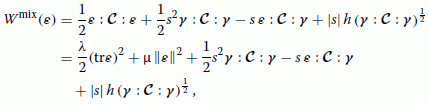(64)

where γ =(m n )s. Herein, $\mathsc{C}$ is the fourth-order isotropic elastic tensor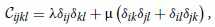(65)

or in the tensor notation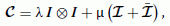(66)

where λ and µ are Lamé constants. The relaxed potential can be defined in the form

$\begin{array}{l}{W}_{R}\left(\mathbit{\epsilon }\right)=\text{inf}\left\{{W}^{\text{mix}}\left(\mathbit{\epsilon }\right)\phantom{\rule{1em}{0ex}}\left|\rights\phantom{\rule{0.3em}{0ex}},\phantom{\rule{0.3em}{0ex}}\mathbit{m}\phantom{\rule{0.3em}{0ex}},\mathbit{n}\phantom{\rule{0.3em}{0ex}};\phantom{\rule{0.3em}{0ex}}∥\mathbit{m}∥=∥\mathbit{n}∥=1\phantom{\rule{1em}{0ex}}\right\}.\end{array}$
(67)

Using the result of Equation 57 we obtain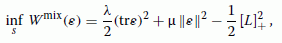(68)

where the quantity L in (62) reduces to

$\begin{array}{l}L=\left[\frac{\left|\mathbit{\epsilon }:\mathsc{C}:\mathbit{\gamma }\right|}{{\left(\mathbit{\gamma }:\mathsc{C}:\mathbit{\gamma }\right)}^{\frac{1}{2}}}-h\right].\end{array}$
(69)

Let φ be the angle between two vectors m and n as depicted in Figure 6, where the unit vector t is perpendicular to the vector m . Then, we may write

$\begin{array}{lcr}\mathbit{n}& =& \mathbit{m}cos\phi +\mathbit{t}sin\phi ,\end{array}$
(70)
$\begin{array}{lcr}\mathbit{m}.\mathbit{\epsilon }\phantom{\rule{0.3em}{0ex}}\mathbit{n}& =& \left(\mathbit{m}.\mathbit{\epsilon }\phantom{\rule{0.3em}{0ex}}\mathbit{m}\right)cos\phi +\left(\mathbit{m}.\mathbit{\epsilon }\phantom{\rule{0.3em}{0ex}}\mathbit{t}\right)sin\mathrm{\phi .}\end{array}$
(71)

Now we consider a plane which has (m ,t ) as the unit tangent and normal vectors. It is recognized that (m .ε m ) is the normal strain whose direction is perpendicular to the plane and (m .ε t ) is the shear strain in this plane. Using Mohr’s circle we can transform (71) into principal strains

$\begin{array}{lcr}\mathbit{m}.\mathbit{\epsilon }\phantom{\rule{0.3em}{0ex}}\mathbit{n}& =& \left({\epsilon }_{m}+Rcos2\psi \right)cos\phi +Rsin2\psi \phantom{\rule{0.3em}{0ex}}sin\phi \\ =& {\epsilon }_{m}cos\phi +Rcos\left(\phi -2\psi \right),\end{array}$
(72)

where R and ε m , respectively, are the maximum shear strain and the average strain

$\begin{array}{l}R=\frac{1}{2}\left({E}_{1}-{E}_{2}\right)\phantom{\rule{1em}{0ex}};\phantom{\rule{1em}{0ex}}{\epsilon }_{m}=\frac{1}{2}\left(\phantom{\rule{1em}{0ex}}{E}_{1}+{E}_{2}\right).\end{array}$
(73)

Herein, ψ is an angle between the vector m and the eigenvector e1 corresponding to the major principal strain E1 as denoted in Figure 7.

Instead of minimizing (67) with respect to s, m and n , now we minimize (74) with respect to s, ψ and φ based on Equations 70 to 73

$\begin{array}{l}{W}_{R}\left(\mathbit{\epsilon }\right)=\text{inf}\left\{{W}^{\text{mix}}\left(\mathbit{\epsilon }\right)\phantom{\rule{1em}{0ex}}\left|\rights\phantom{\rule{0.3em}{0ex}},\phantom{\rule{0.3em}{0ex}}\psi \phantom{\rule{0.3em}{0ex}},\phi \phantom{\rule{0.3em}{0ex}};\phantom{\rule{0.3em}{0ex}}0\le \psi \phantom{\rule{0.3em}{0ex}},\phantom{\rule{0.3em}{0ex}}\phi \le \pi \phantom{\rule{1em}{0ex}}\right\}.\end{array}$
(74)

The minimization of (74) with respect to s, ψ and φ yields

R=0:

$\begin{array}{l}\psi =\phi =0,\end{array}$
(75)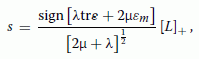(76)

where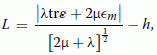(77)

R≠0: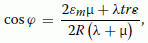(78)
$\begin{array}{l}\psi =\frac{\phi }{2},\end{array}$
(79)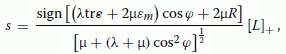(80)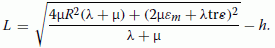(81)

The capability of the proposed model is demonstrated through numerical simulation of a tension test in the next section.

### Example 2

In this section, the model presented in section ‘Relaxed potential’ are implemented into the finite element code FEAP . In what follows, we investigate a tension test under plane strain conditions. The main goal of the study is the analysis of the developing shear bands and the demonstration of mesh independent results due to the proposed relaxation technique. Two kinds of elements are used in this example as summarized in Figure 8.

A sample is subjected to a prescribed vertical displacement under plane strain conditions. The geometry of the specimen, the boundary conditions and the material parameters are given in Figure 9. $h=1\sqrt{N}/\mathit{\text{mm}}$. In order to trigger the shear band formation, a geometrical imperfection along the height of specimen is introduced.

Four discretizations of the domain are considered: 3 × 8, 7 × 18, 14 × 36, 21 × 54 elements. The response obtained using the four different meshes is the same with respect to load-displacement curves as shown in Figure 10a, thus verifying the lack of pathological mesh-dependence of the proposed concept. As soon as the onset of localization is met at v≈0.2427 m m which signals the loss of convexity, the performance of the diverse element formulations employed starts to differ.

The displacement method is unable to capture the localization and shows a hardening behaviour as depicted in Figure 10a,b.

However, Figure 11 demonstrates that the mixture element formulation can resolve the effect of strain localization. While in Figure 11a,b, the mesh is still too coarse to exhibit shear bands, these are clearly represented in a mesh-independent way in Figure 11c,d. There exist two symmetric shear bands in this example and their orientations are about 60° and 120° with respect to the horizontal axis.

The behaviour of the relaxed potential as well as the relaxed vertical stress σ y of the element 465 (Figure 11e) is shown in Figure 12 at the first Gauss point inside the shear band. After the bifurcation point, the relaxed potential of the low-strain domain approaches asymptotically a constant value, whereas the relaxed potential of the high-strain domain is active inside the shear band only and increases continuously with the prescribed displacement v. As a result, the relaxed stress approaches a constant value.

## Application of relaxation theory to inelastic materials

### Relaxed potential

For simplicity we consider the special case $\mathsc{D}={A}^{2}\mathsc{I}$ in this section with assuming orthogonality, i.e. m .n =0 of the two unit vectors giving the direction of shear band evolution. Herein, A is a material parameter and $\mathsc{I}$ is the fourth-order unity tensor. Furthermore, we assume that evolution of these two vectors over time is not remarkable.

We assume that the elastic deformation is small compared to the inelastic deformation and can be neglected, yielding Equation 5:

$\begin{array}{l}{\epsilon }_{\mathrm{I}}={\nabla }^{\mathrm{s}}\mathbit{u}.\end{array}$
(82)

Together with the above assumptions, the mixture dissipation potential can be obtained with s replaced by $\stackrel{̇}{s}$ and Wmix(ε ) by ${\Delta }^{\text{mix}}\left({\stackrel{̇}{\epsilon }}_{\mathrm{I}}\right)$ in Equation 53

$\begin{array}{lcr}{\Delta }^{\text{mix}}\left({\stackrel{̇}{\mathbit{\epsilon }}}_{\mathrm{I}}\right)& =& \frac{1}{2}\left({\stackrel{̇}{\mathbit{\epsilon }}}_{\mathrm{I}}-\stackrel{̇}{s}\mathbit{\gamma }\right):\mathsc{C}:\left({\mathbit{\epsilon }}_{\mathrm{I}}-\stackrel{̇}{s}\mathbit{\gamma }\right)+\frac{A}{\sqrt{2}}\left|\stackrel{̇}{s}\right|\end{array}$
(83)

where γ =(m n )s. Let us denote by ${\Delta }_{1}^{\text{mix}}$ and ${\Delta }_{2}^{\text{mix}}$

$\begin{array}{lcr}{\Delta }_{1}^{\text{mix}}& =& \frac{1}{2}\left({\stackrel{̇}{\mathbit{\epsilon }}}_{\mathrm{I}}-\stackrel{̇}{s}\mathbit{\gamma }\right):\mathsc{C}:\left({\mathbit{\epsilon }}_{\mathrm{I}}-\stackrel{̇}{s}\mathbit{\gamma }\right),\end{array}$
(84)
$\begin{array}{lcr}{\Delta }_{2}^{\text{mix}}& =& \frac{A}{\sqrt{2}}\left|\stackrel{̇}{s}\right|,\end{array}$
(85)

then, the mixture dissipation potential Δmix is the sum of ${\Delta }_{1}^{\text{mix}}$ and ${\Delta }_{2}^{\text{mix}}$.

By substituting (1) into (83), the relaxed potential reads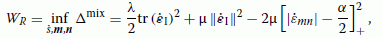(86)

where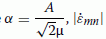is the maximum shear strain rate.

Solution $\stackrel{̇}{s}$ yields

$\begin{array}{l}\stackrel{̇}{s}={\left(2\left|{\stackrel{̇}{\epsilon }}_{\mathit{\text{mn}}}\right|-\alpha \right)}_{+}\text{sign}\left({\stackrel{̇}{\epsilon }}_{\mathit{\text{mn}}}\right)=\left\{\begin{array}{ll}0& \text{if}\phantom{\rule{0.3em}{0ex}}\left|{\stackrel{̇}{\epsilon }}_{\mathit{\text{mn}}}\right|<\alpha /2\\ \left(2\left|{\stackrel{̇}{\epsilon }}_{\mathit{\text{mn}}}\right|-\alpha \right)\text{sign}\left({\stackrel{̇}{\epsilon }}_{\mathit{\text{mn}}}\right)& \text{if}\phantom{\rule{0.3em}{0ex}}\left|{\stackrel{̇}{\epsilon }}_{\mathit{\text{mn}}}\right|\ge \alpha /2\end{array}\right\\end{array}$
(87)

Unlike elastic materials, inelastic materials can be incorporated as well via a time-incremental formulation

$\begin{array}{l}{W}_{R,n+1}={W}_{R,n}+\Delta {W}_{R}\end{array}$
(88)

where WR,n+1 and WR,n, respectively, are the potential energies at times tn+1 and t n , Δ W is the incremental potential at time interval Δ t

$\begin{array}{l}\Delta {W}_{R}=\underset{{t}_{n}}{\overset{{t}_{n+1}}{\int }}{W}_{R}\phantom{\rule{0.3em}{0ex}}\mathrm{dt.}\end{array}$
(89)

The proposed formulation is implemented in the general code FEAP . Based on the mixed enhanced strain method , the four-node quadrilateral element (MES element) will be considered in the next section.

### Example 3

A sample is subjected to vertical loading and unloading under plane strain conditions as shown in Figure 13. A geometrical imperfection along the height of specimen is introduced to trigger the shear band formation.

Four discretizations of the domain are considered: 828, 2,146, 2,310 and 2,480 elements. As depicted in Figure 14, mesh-independence response obtained by use of MES element becomes evident by considering load-displacement diagram at the top of the specimen. The distributions of the horizontal displacement and of the relaxed energy shown in Figure 15 point out the localized region. The relaxed energy and the relaxed vertical stress of the element 956 at the first Gauss point are shown in Figure 16. The relaxed potential of the large-strain domain ${\left({\Delta }_{2}^{\text{mix}}\right)}_{\mathrm{R}}$ develops linearly between ε y =0.035 and ε y =0.095 (2.7 st≤2.73 s), then remains constant when ε y ≥0.095 corresponding to the unloading path. The relaxed stress is also constant for 0.035≤ε y ≤0.095, and it diminishes after ε y ≥0.095 due to the prescribed unloading.

## Conclusions

The paper focuses on a theoretical framework for the treatment of shear localization in solid materials. The theory is based on minimization principles associated with micro-structure developments under the assumptions of a micro-shear band of a zero thickness and the presence of a mixture potential inside the shear band.

Localization phenomena are regarded as micro-structure developments associated with nonconvex potentials. The nonconvexity of the mixture potential occurring due to the formation of strain localization is resolved by relaxation in order to ensure the well-posedness of the associated boundary value problem. The relaxed potential, which is approximated by a first-order rank-one convexification, is obtained via local minimization problem of the mixture potential. The onset of localization is detected through the proposed optimization process. The model can be applied to any material which softens towards the critical state. The relaxed stress can be computed directly and approaches the critical stress as soon as strain localization occurs. Material points located inside the shear bands can be considered as decomposed into a low strain and a high strain phase at the microscopic level. The theoretical solutions satisfy possessing a zero micro-band width at the microscopic level. At the macroscopic scale, the width of a shear band is still finite. Numerical results clearly show a mesh-independent behaviour in the sense that shear bands are as narrow as the mesh resolution allows, while all other features of the solution are independent of the chosen discretization.

## References

1. Borja R: A finite element model for strain localization analysis of strongly discontinuous fields based on standard Galerkin approximation. Comput Methods Appl Mech Engrg 2000, 190: 1529–1549. 10.1016/S0045-7825(00)00176-6

2. Lott-Crumpler DA: The formation and properties of shear bands in viscoplastic materials. Model Simul Mater Sc 1997, 5: 317–336. 10.1088/0965-0393/5/4/002

3. Engelen RAB, Geers MGD, Baaijens FPT: Nonlocal implicit gradient-enhanced elasto-plasticity for the modelling of softening behaviour. Int J Plasticity 2003, 19: 403–433. 10.1016/S0749-6419(01)00042-0

4. Mühlhaus HB, Vardoulakis I: The thickness of shear bands in granular materials. Géotechnique 1987, 37: 271–283. 10.1680/geot.1987.37.3.271

5. de Borst R, Sluys LJ: Localization in a Cosserat continuum under static and dynamics loading conditions. Comput Methods Appl Mech Engrg 1991, 90: 805–827. 10.1016/0045-7825(91)90185-9

6. Huang W, Bauer E: Numerical investigations of shear localization in a micro-polar hypoplastic material. Int J Numer Anal Methods Geomech 2003, 27: 325–352. 10.1002/nag.275

7. Strömberg L, Ristinmaa M: FE-formulation of a non-local plasticity theory. Comput Methods Appl Mech Engrg 1996, 136: 127–144. 10.1016/0045-7825(96)00997-8

8. Baz̆ant ZP, Belytschko TB, Chang TP: Continuum model for strain softening. J Eng Mech (ASCE) 1984, 110: 1666–1692. 10.1061/(ASCE)0733-9399(1984)110:12(1666)

9. de Borst R, Mühlhaus HB: Gradient-dependent plasticity: formulation and algorithmic aspects. Int J Numer Meth Eng 1992, 35: 521–539. 10.1002/nme.1620350307

10. Pamin J: Gradient-dependent plasticity in numerical simulation of localization phenomena. Delft University of Technology, The Netherlands; 1994.

11. Larsson R, Steinmann P, Runesson K: Finite element embedded localization band for finite strain plasticity based on a regularized strong discontinuity. Mech Cohes-Frict Mat 1998, 4: 171–194. 10.1002/(SICI)1099-1484(199903)4:2<171::AID-CFM81>3.0.CO;2-X

12. Simo JC, Oliver J, Armero F: An analysis of strong discontinuities induced by strain-softening in rate-independent inelastic solids. Comput Mech 1993, 12: 277–296. 10.1007/BF00372173

13. Oliver J: Modelling strong discontinuities in solid mechanics via strain softening constitutive equations: fundamental and numerical simulation. Monograph CIMNE No. 28. Internacional Center for Numerical Methods in Engineering, Barcelona; 1995.

14. Armero F, Garikipati K: An analysis of strong discontinuities in multiplicative finite strain plasticity and their relation with the numerical simulation of strain localization in solids. Int J Solids Struct 1996, 33: 2863–2885. 10.1016/0020-7683(95)00257-X

15. Steinmann P, Larsson R, Runesson K: On the localization properties of multiplicative hyperelasto-plastic continua with strong discontinuities. Int J Solids Struct 1997, 34: 969–990. 10.1016/S0020-7683(96)00043-1

16. Pietruszczak S, Xu G: Brittle response of concrete as a localization problem. Int J Solids Struct 1995, 32: 1517–1533. 10.1016/0020-7683(94)00231-K

17. Xu G, Pietruszczak S: Numerical analysis of concrete fracture based on a homogenization technique. Comput Struc 1997, 63: 497–509. 10.1016/S0045-7949(96)00304-5

18. Armero F: Large-scale modeling of localized dissipative mechanisms in a local continuum: applications to the numerical simulation of strain localization in rate-dependent inelastic solids. Mech Cohes-Frict Mater 1999, 4: 101–131. 10.1002/(SICI)1099-1484(199903)4:2<101::AID-CFM78>3.0.CO;2-Y

19. Nguyen GD, Einav I, Korsunsky AM: How to connect two scales of behaviour in constitutive modelling of geomaterials. Geotech Lett 2012, 2: 129–134. 10.1680/geolett.12.00030

20. Nguyen GD, Einav I, Korsunsky AM: A constitutive modelling framework featuring two scales of behaviour: Fundamentals and applications to quasi-brittle failure. Eng Fract Mech 2012, 115: 221–240. 10.1016/j.engfracmech.2013.11.006

21. Hackl K, Hoppe U (2001) On the calculation of microstructures for inelastic materials using relaxed enegies. In: Miehe C (ed)Proceedings of the IUTAM Symposium on Computational Mechanics of Solid Materials at Large Strains, 77–86, Boston. Hackl K, Hoppe U (2001) On the calculation of microstructures for inelastic materials using relaxed enegies. In: Miehe C (ed)Proceedings of the IUTAM Symposium on Computational Mechanics of Solid Materials at Large Strains, 77–86, Boston.

22. Ball JM, James RD: Fine phase mixtures as minimizer of energy. Arch Ration Mech Ana 1987, 100: 13–52. 10.1007/BF00281246

23. Dacorogna B: Direct methods in the calculus of variations. Springer-Verlag, Berlin; 1989.

24. Kohn R: The relaxation of a double-well energy. Continuum Mech Therm 1991, 3: 193–236. 10.1007/BF01135336

25. Bartel S, Carstensen C, Hackl K, Hoppe U: Effective relaxation for microstructure simulations: algorithms and applications. Comput Methods Appl Mech Engrg 2004, 193: 5143–5175. 10.1016/j.cma.2003.12.065

26. Hackl K, Heinen R: A micromechanical model for pretextured polycrystalline shape-memory alloys including elastic anisotropy. Continuum Mech Therm 2008, 19: 499–510. 10.1007/s00161-008-0067-z

27. Carstensen C, Hackl K, Mielke A: Non-convex potentials and microstructures in finite-strain plasticity. Proc R Soc Lond A 2002, 458: 299–317. 10.1098/rspa.2001.0864

28. Hackl K, Fischer FD: On the relation between the principle of maximum dissipation and inelastic evolution given by dissipation potentials. Proc R Soc Lond A 2008, 464: 117–132. 10.1098/rspa.2007.0086

29. Lambrecht M, Miehe C, Dettmar J: Energy relaxation of non-convex incremental stress potentials in a strain-softening elastic-plastic bar. Int J Solids Struct 2003, 40: 1369–1391. 10.1016/S0020-7683(02)00658-3

30. Miehe C, Lambrecht M: A two-scale finite element relaxation analysis of shear bands in non-convex inelastic solids: small-strain theory for standard dissipative materials. Comput Methods Appl Mech Engrg 2003, 192: 473–508. 10.1016/S0045-7825(02)00547-9

31. Miehe, C, Lambrecht, M: Analysis of microstructure development in shearbands by energy relaxation of incremental stress potentials: large-strain theory for standard dissipative solids Int J Numer Meth Eng 2003, 58: 1–41. 10.1002/nme.726

32. Miehe C, Lambrecht M, Guerses E: Analysis of microstructure development in shearbands by energy relaxation of incremental stress potentials: large-strain theory for standard dissipative solids. J Mech Phys Solids 2004, 52: 2725–2769. 10.1016/j.jmps.2004.05.011

33. Guerses E, Miehe C: On evolving deformation microstructures in non-convex partially damaged solids. J Mech Phys Solids 2011, 59: 1268–1290. 10.1016/j.jmps.2011.01.002

34. Mistakidis ES: On the solution of structures involving elements with nonconvex energy potentials. Struct Optimization 1997, 13: 182–190. 10.1007/BF01199238

35. Kochmann DM, Hackl K: The evolution of laminates in finite plasticity: a variational approach. Continuum Mech Thermodyn 2011, 23: 63–85. 10.1007/s00161-010-0174-5

36. Trinh BT, Hackl K: Finite element simulation of strain localization in inelastic solids by energy relaxation. Vietnam J Mech 2011, 33: 203–213.

37. Mielke A: Deriving new evolution equations for microstructures via relaxation of variational incremental problems. Comput Methods Appl Mech Engrg 2004, 193: 5095–5127. 10.1016/j.cma.2004.07.003

38. de Borst R: Damage, material instabilities, and failure, Chapter 10. In Encyclopedia of Computational Mechanics, vol. 2. Edited by: Stein E, de Borst R, Hughes TJR. Wiley, Chichester; 2004:335–373.

39. Kohn RV, Strang G: Optimal design and relaxation of variational problems I, II, III. Comm Pure Appl Math 1986, 39: 113–137. 10.1002/cpa.3160390107

40. Hencky H: Zur Theorie plastischer Deformation und der hierdurch im Material hervorgerufenen Nachspannungen. Z Angew Nath Mech 1924, 4: 323–333. 10.1002/zamm.19240040405

41. Ilyushin AA: Some problems of plastic deformations. PMM 1943, 7: 245–272.

42. Ilyushin AA: Theory of plasticity at simple loading of the bodies exhbiting plastic hardening. PMM 1947, 11: 291–296.

43. Gao Y, Huang K: Complementary energy principles for elasto-perfectly plasticity. Scientia Sinica 1988, 31: 1469–1476.

44. Budhu M: Soil mechanics and foundations. United States of America, Wiley; 2000.

45. Taylor RL (2004) A finite element analysis program: User manual, University of California at Berkeley. Taylor RL (2004) A finite element analysis program: User manual, University of California at Berkeley.

46. Kasper EP, Taylor RL (1997) A mixed-enhanced strain method: linear problems. Report No. UCB/SEMM-97/02, University of California at Berkeley. Kasper EP, Taylor RL (1997) A mixed-enhanced strain method: linear problems. Report No. UCB/SEMM-97/02, University of California at Berkeley.

47. Zienkiewicz OC, Taylor RL: The finite element method. Volume 1: The Basis (5nd edn). Butterworth-Heinmann, London; 2000.

## Acknowledgements

The research was supported through grants by the Vietnamese Government and Ministry of Education and Training as well as the Research School at Ruhr University Bochum, Germany.

## Author information

Authors

### Corresponding author

Correspondence to Tuyet B Trinh.

### Competing interests

The authors declare that they have no competing interests.

### Authors’ contributions

TT and KH contributed in equal measure. Both authors read and approved the final manuscript.

## Authors’ original submitted files for images

Below are the links to the authors’ original submitted files for images.

## Rights and permissions